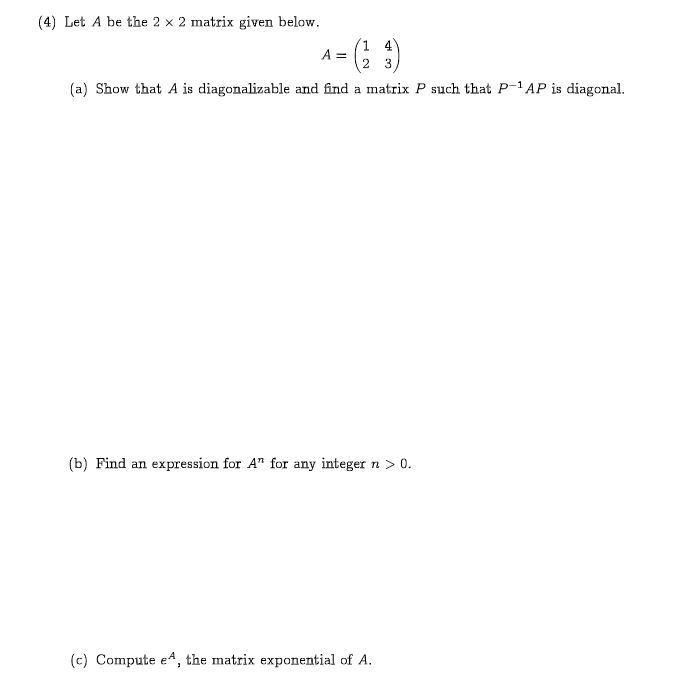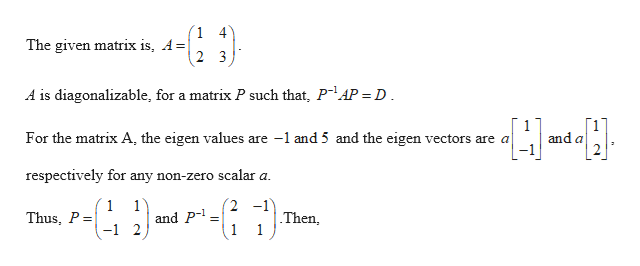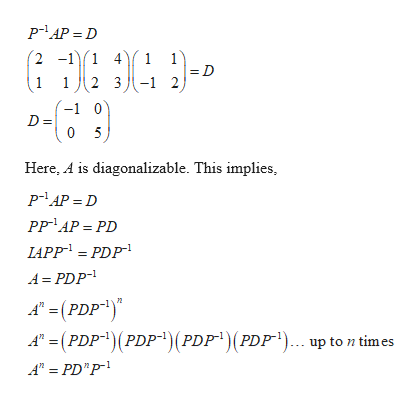# (4 Let A be the 2 x 2 matrix given below1 4A =23(a) Show that A is diagonalizable and find a matrix P such that P-1AP is diagonal.(b) Find an expression for A" for any integer n > 0.(c) Compute eA, the matrix exponential of A.

Question
1 viewshelp_outlineImage Transcriptionclose(4 Let A be the 2 x 2 matrix given below 1 4 A = 2 3 (a) Show that A is diagonalizable and find a matrix P such that P-1AP is diagonal. (b) Find an expression for A" for any integer n > 0. (c) Compute eA, the matrix exponential of A. fullscreen
check_circle

Step 1help_outlineImage Transcriptionclose1 4 The given matrix is, A| 2 3 A is diagonalizable, for a matrix P such that, P-1AP = D 1 and a For the matrix A, the eigen values are -1 and 5 and the eigen vectors are a respectively for any non-zero scalar a (2 -1 1 Thus, P = 1 and P Then, 1 -1 2 1 fullscreen
Step 2help_outlineImage Transcriptionclose2 -1)1 4 1 1 2 3-1 2 1 -1 0 D = 0 5 Here, A is diagonalizable. This implies PP AP PD LAPP = PDP A PDP A(PDP) APDP(PDP A PD" P (PDF") (PDF ).. up to n times fullscreen

### Want to see the full answer?

See Solution

#### Want to see this answer and more?

Solutions are written by subject experts who are available 24/7. Questions are typically answered within 1 hour.*

See Solution
*Response times may vary by subject and question.
Tagged in

### Math